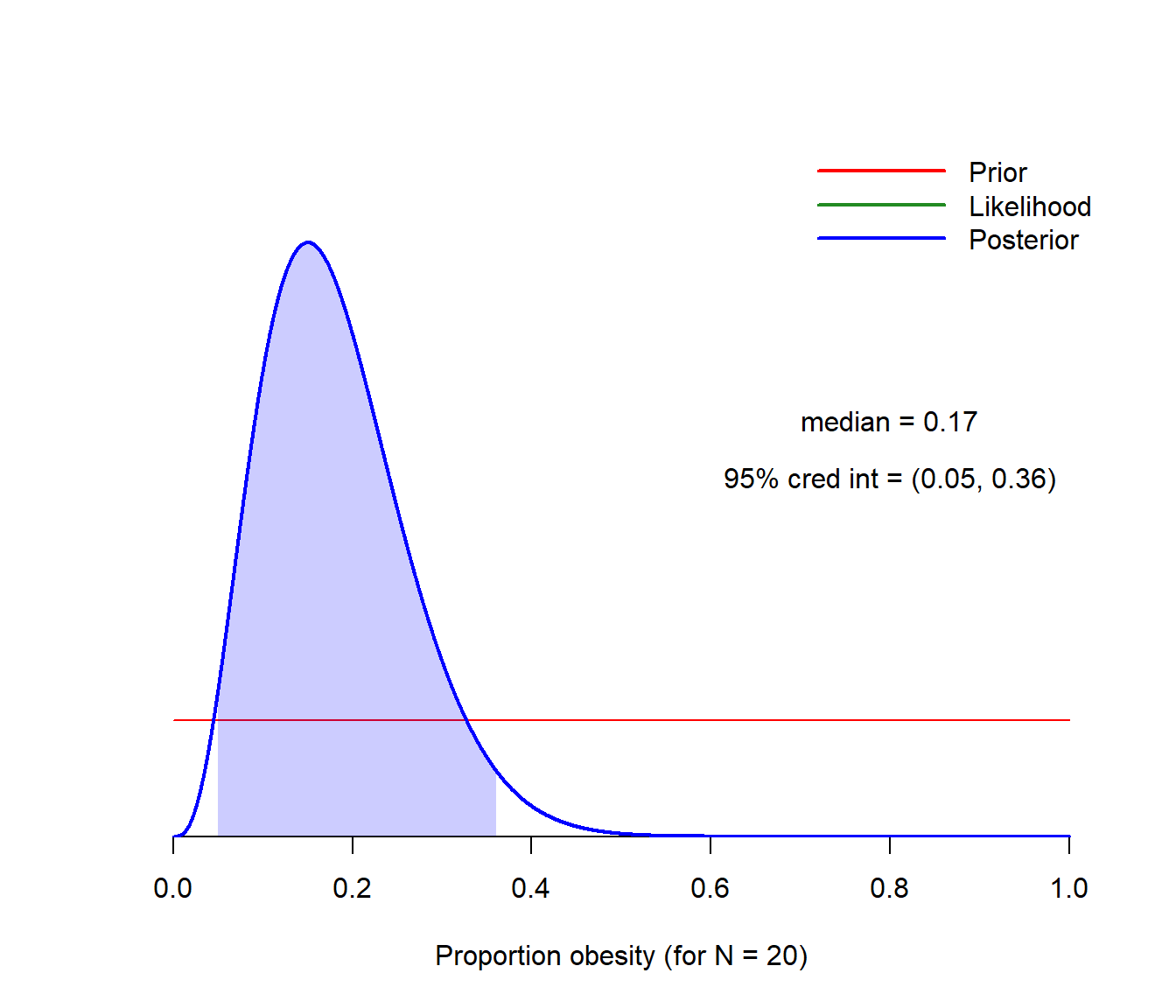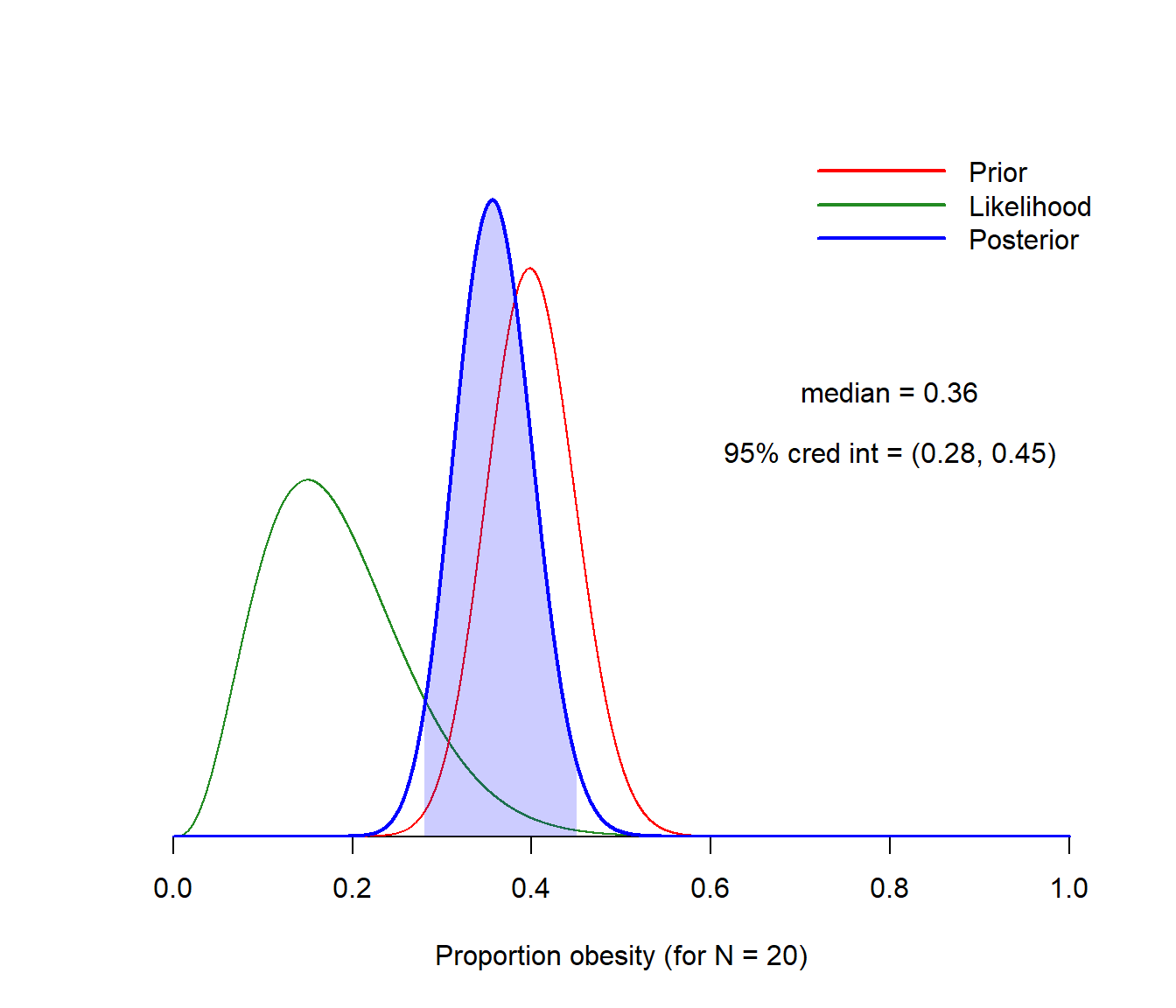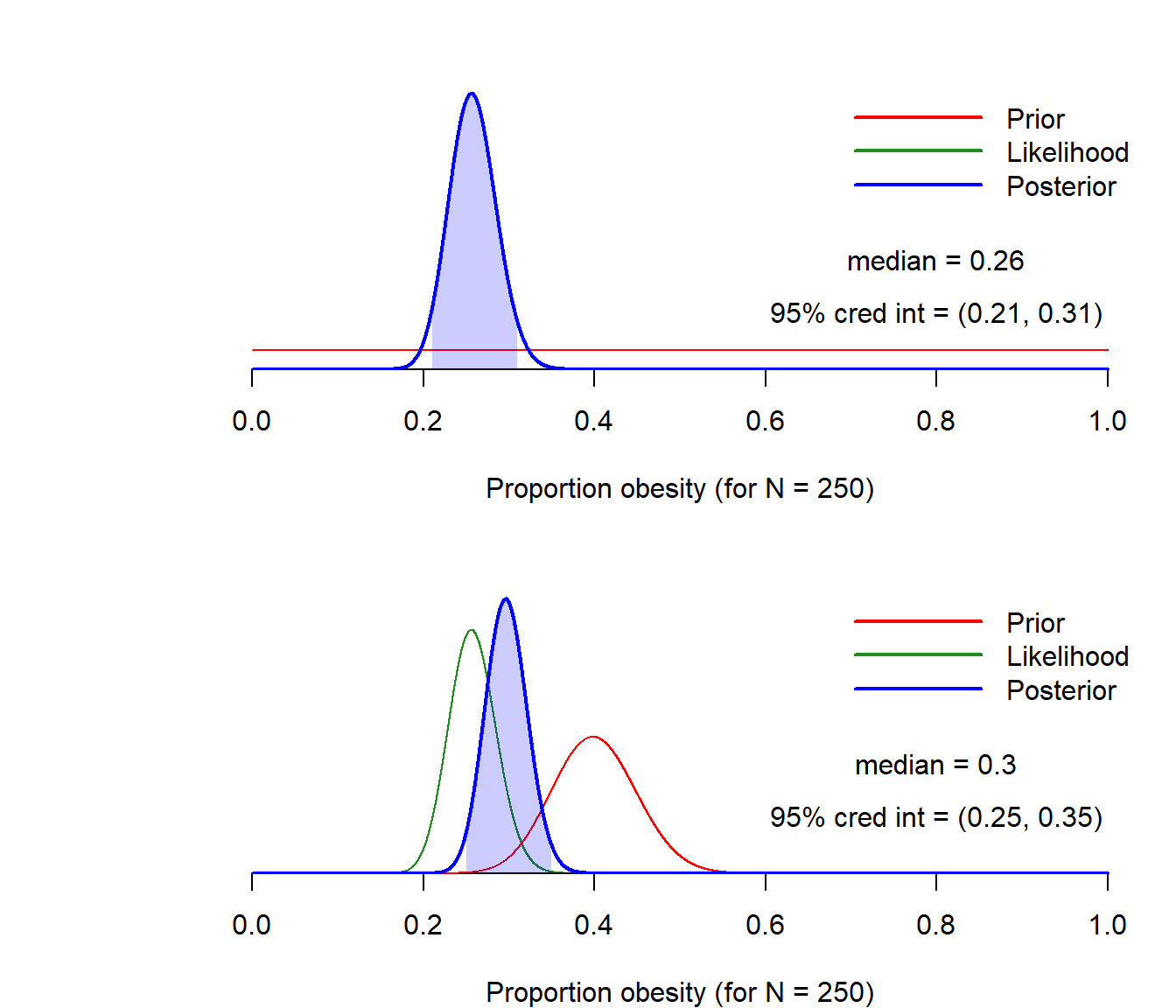# 1 Basics

## 1.1 Bayes rule

Say you have data $$\mathcal{D}$$, which you use to improve your understanding on the possible values of an unknown parameter $$\theta$$. For instance, $$\theta$$ can be the proportion of obese men in a particular population, where male obesity is operationalized as percentage of body fat (PBF) above 25%. Data $$\mathcal{D}$$ are the PBF scores for a sample of men from that population.

Bayesian statistics works as follows:

• Select a statistical model. The model conceptualizes how theory and data meet: It expresses, for any given value of the model parameter $$\theta$$, what the probability of any observed data is. This is denoted by $$p(\mathcal{D}|\theta)$$ (read: “probability of $$\mathcal{D}$$ conditional on a particular $$\theta$$ value”) and often referred to as the likelihood function.

• Before looking at the data, express your belief about the value of $$\theta$$ in the population. This ‘belief’ does not equate with the ‘religious’ kind of belief. Instead, it refers to an educated expression of one’s knowledge about the world. For instance, it is possible that previous research found proportions of male obesity typically between .15 and .30. This knowledge can (and should!) be incorporated in the analysis. The prior distribution, denoted $$p(\theta)$$, does exactly this.

• By means of the Bayes rule (see below), update your knowledge. Before the experiment, your knowledge about $$\theta$$ is encoded by the prior distribution. After looking at the data, you will rationally update your belief about $$\theta$$. The so-called posterior distribution, here denoted $$p(\theta|\mathcal{D})$$, represents your updated knowledge.

The Bayes rule can be written as follows:

$p(\theta|\mathcal{D}) = \frac{p(\theta)p(\mathcal{D}|\theta)}{p(\mathcal{D})}.$

It doesn’t look nice…

Perhaps rewriting Bayes rule in words helps:

$\text{posterior} = \frac{\text{prior} \times \text{likelihood}}{\text{evidence}}.$

Notice that the evidence, $$p(\mathcal{D})$$, does not depend on $$\theta$$. It is there just to ensure that the posterior distribution is well defined (among other things that we won’t be discussing today). For most purposes we can actually hide it and rewrite Bayes rule like this:

$\text{posterior} \propto \text{prior} \times \text{likelihood},$ where $$\propto$$ means “proportional to”.

Therefore, the posterior distribution is basically a (rational, logically correct) means of merging together both our prior knowledge about some phenomenon with the information about the phenomenon that our data has to offer.

## 1.2 A small example

Let’s make things concrete. I downloaded data from https://dasl.datadescription.com/datafile/bodyfat/, containing various measurements of 250 men. I focus on variable ‘Pct.BF’ (percentage of body fat) and dichotomize it (0 = PBF lower than 25%; 1 = PBF larger than 25%). I want to infer the proportion of obese men in the population.

PBF.data <- read.csv(url("https://dasl.datadescription.com/download/data/3079"),
header = TRUE, sep = "\t")
PBF      <- ifelse(PBF.data\$Pct.BF > 25, 1, 0)
head(PBF)
##  0 0 1 0 1 0
length(PBF)
##  250
prop.table(table(PBF))
## PBF
##     0     1
## 0.744 0.256

Let’s start by focusing on the data from the first 20 subjects in the sample. Suppose, rather unrealistically, that a priori all proportions of obese men in the population are equally likely. Using the binomial model to account for the number of obese men in the sample, the three elements of Bayesian statistics (prior, likelihood, posterior) can be visualized as follows:Observe that:

• The prior expresses the fact that all proportions are equally likely, before taking the data into account.

• The posterior distribution expresses our updated knowledge about the population proportion, conditional on our model and data.

• The likelihood (rescaled to unit area) coincides with the posterior distribution in this case of a uniform prior, and that is why it appears to be missing.

Let’s improve the prior distribution. Suppose we expected, a priori, that the proportion of obese men in the population would be about 40%. We can pick a prior which peakes around .4 and redo the computations:The posterior distribution changed, as it should: Changes in the prior and/or likelihood will lead to changes in the posterior. Observe how the posterior distribution is a balance between the information in the data (the likelihood) and the information a priori (the prior). Bayesian statistics is all about rendering a compromise between these two sources.

Above I displayed two estimated models, rendering two rather different versions of updated knowledge about the proportion of obesity. The data are the same, yet the conclusions differ… This reflects uncertainty related to the model. What happens if we have more data? Let’s reproduce the two previous plots, this time using the scores of all 250 men in the sample:As can be seen, even though the priors differ a lot, the posteriors now look more similar. The reason is that there is typically more information in a sample of size 250 than in a sample of size 20. As the information provided by the data accummulates, the updated knowledge tends to depend less and less on the prior. In this way, it is possible that two persons with very dissimilar starting points (=priors) end up with a similar updated knowledge (=posteriors) about the phenomenon at hand.

Why are posterior distributions useful? In essence, they can provide direct answers to research questions. For example, based on the posterior plotted on the bottom panel above:

• What is the posterior probability that the population proportion of obese men is larger than 30%?